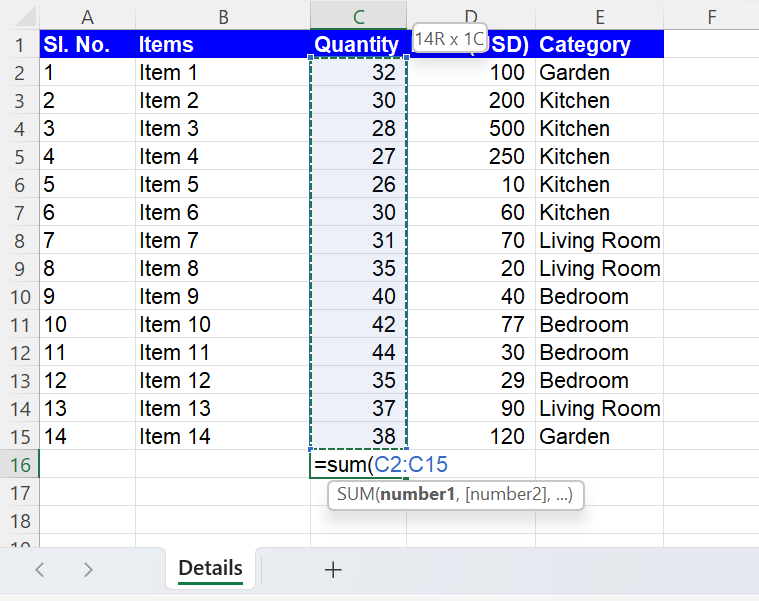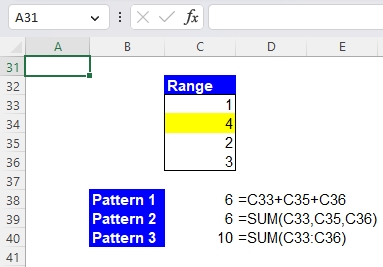Excel Formulas

# SUM Function in Excel: Explained

In this article, you will learn how to use the SUM formula in Excel.

## What is the SUM function in Excel?

In Microsoft Excel, the SUM function adds together a range of cells.

## When is the SUM formula useful in Excel?

The SUM formula in Excel is beneficial in various situations when you need to sum up a range of values. Examples include:

1. Totaling up a column or row of numbers: For example, if you have a column of sales figures for a month and want to know the total monthly sales, you can use the SUM formula to add them all up.
2. Financial analysis: In financial analysis or accounting, you may want to find the total revenue, total expenses, total profit, etc. the SUM formula can be used to add together the values for each category.

## How to use the SUM function in Excel

The syntax for the function is as follows:

``=SUM(number1,[number2],...)``

Each of the "number" arguments can be a number, such as “10”, a cell, such as “A1”, a range of cells, such as "A1:A10", or a named range, such as "sales". You can enter up to 255 arguments by dividing each parameter by commas. The function will add all values within the specified range and return the sum.

As described above, you can use the manual inputs to use the SUM function, such as below.

``=SUM(1,2,3)``

Instead, you can use cell references to enter the argument(s) in the SUM formula. For example, if cells B4, C4, and D4 contain the values 1, 2, and 3, respectively, the following formula will return the value 6.

``=SUM(B4,C4,D4)``

Also, the following formulas return the same value as shown in the screenshot below.

``=SUM(B4,C4:D4)``
``=SUM(B4:C4,D4)``
``=SUM(B4:D4)``

## Inserting the SUM function in Excel

To further understand the SUM formula better, follow the below step-by-step instructions on inserting the SUM function.

Step 1: Type “=SUM” in the cell where you want to add values

Step 2: Once the formula prompt opens up, click on the SUM formula

Step 3: Select the values one by one by separating them with “,” (commas). You can also select an array of data as shown in the image below.Image showing the SUM formula used to select an array of data in Excel

Step 4: Press the “ENTER” key

## How to use the AutoSum function in Excel

Alternatively, you can also use the AutoSum function to aggregate a range of cells.

1. Select the cell where you want the sum to appear, or choose the range you want to add.
2. Press “Alt” + “=” (Hold down the “Alt” key and press the “equal” key), or navigate to the “Formula” tab, click the “AutoSum” button, and select “SUM”.
3. Press Enter, and it will automatically select the cells above or the left of the selected cell, depending on where you positioned the cursor, or show the result in a cell below or right to the selected range.

## What are the best practices with the SUM formula in Excel?

In this section, you can learn essential points you need to bear in mind when using the SUM function in Excel. Most of these tips can be applied to other formulas.

Manual Input vs. Cell Reference

As we explained above, you can manually input numeric values in the formula to add them up. However, you should refrain from doing so when you need to calculate numbers with long digits or many decimal places, as there is a possibility of a typo(s) leading to a wrong calculation result. It is recommended to input those numbers into cells (manually), making it easier for you to double-check the values and then sum them up with the SUM function by cell reference.

#VALUE! error value

Look at the following screenshot. As you can see, if you conduct addition without the SUM function (Pattern 1), when one of the added cells contains a non-numeric value (text), the formula returns #VALUE! Error. On the other hand, if you use the SUM formula (Pattern 2 and 3) and aggregate the same range of cells, the formula returns the total of numerical values in the range.

#REF! Error value (Individual Cell Reference vs. Range Reference)

Assume cells C24, C25, and C26 contain 1, 2, and 3, respectively, and you have three types of formulas; (Pattern 1) =C24+C25+C26; (Pattern 2) =SUM(C24,C25,C26); and (Pattern 3) =SUM(C24:C26). What happens to these formulas when you delete row 25? The formulas with individual cell references (Pattern 1 and 2) are not updated and return #REF! Error. To fix the issue, you need to take out the #REF! Values from the formulas. On the other hand, the SUM formula referring to a range is automatically updated and still returns the total value of the selected range. Thus, using the SUM with range reference is recommended when you may delete a row(s) or column(s), which is a part of the calculation. The following screenshot shows the three patterns of the formula after removing Row 25, in which the original Row 26 changed to Row 25.

Automatic formula update (Individual Cell Reference vs. Range Reference)

Assume cells C33, C34, and C35 contain 1, 2, and 3, respectively, and you have three types of formulas; (Pattern 1) =C33+C34+C35; (Pattern 2) =SUM(C33,C34,C35); and (Pattern 3) =SUM(C33:C35). What happens to these formulas when you insert a row between Rows 33 and 34 and put a new number there? The answer is that Parttens 1 and 2 still only refer to the original cells (C33, C35 (former C34), and C36 (former C35) and show the same computation results even after the new row is inserted, and the new number is entered. However, as shown in the screenshot below, Pattern 3 is automatically updated. Pattern 3 is recommended when you may insert a new row(s) or column(s), which contains the value you want to sum up together with the original values.How to update the SUM function automatically when a new row or column is inserted in Excel

## Analyze your live financial data in a snap in Google Sheets

Are you learning this formula to visualize financial data, build a financial model, or conduct financial analysis? In that case, LiveFlow may help you automate manual workflows, update numbers in real-time, and save time. You can access various financial templates on our website, from the simple Income Statement to Multi-Currency Consolidated Financial Statement. Are you interested in this product but are an Excel user? That’s not a problem at all. You can connect Google Sheets to Excel quickly.